1. Introduction

In this tutorial we will make choropleth maps, sometimes called heat maps, using the ggplot2 package. A choropleth map is a map that shows a geographic landscape with units such as countries, states, or watersheds where each unit is colored according to a particular value.

We start by using requiring three packages, ggplot2, dplyr, and maps. The maps package simply contains several simple data files that allow us to create maps. Before working through this tutorial, you should be familiar with basic ggplot and dplyr functions.

library(ggplot2)  # The grammar of graphics package
library(maps)     # Provides latitude and longitude data for various maps
library(dplyr)    # To assist with cleaning and organizing data

Data: In addition to data files from the maps package, we will use the StatePopulation data, which includes the state name, estimated population, and the number of electoral college votes each state is allocated.

# load United States state map data
MainStates <- map_data("state")

# read the state population data
StatePopulation <- read.csv("C:/Users/KUIPERS/Desktop/RTutorials/MakingMapsIntro/StatePopulation.csv", as.is = TRUE)
# str(MainStates)
# str(StatePopulation)

2. Making a base map

Creating maps follows the same grammar of graphics structure as all other ggplots. Here we use the appropriate dataset (MainStates), geom(polygon), and aesthetics (latitude and longitude values) to create a base map.

#plot all states with ggplot2, using black borders and light blue fill
ggplot() +
geom_polygon( data=MainStates, aes(x=long, y=lat, group=group),
color="black", fill="lightblue" )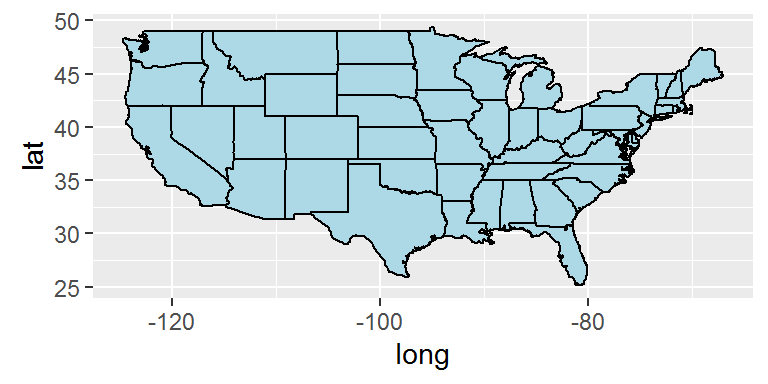Questions:
On page two of the maps package documentation, we see that in addition to state, the maps package includes county, france, italy, nz, usa, world and world2 files.

1. Create a base map of United States counties. Make sure you use the map_data function as well as the ggplot function.

2. Use the world file to create a base map of the world with white borders and dark blue fill.

3. Notice that the MainStates file has a column titled “group”. What happens to your base map when group is ignored (i.e. run the code ggplot() + geom_polygon( data=MainStates, aes(x=long, y=lat)))?

3. Customizing your choropleth map

Now that we have created a base map of the mainland states, we will color each state according to its population. The first step is to use the dplyr package to merge the MainStates and StatePopulation files.

# Use the dplyr package to merge the MainStates and StatePopulation files
MergedStates <- inner_join(MainStates, StatePopulation, by = "region")
# str(MergedStates)

Next we create a base map of the mainland United States, using state population as a fill variable.

# Create a Choropleth map of the United States
p <- ggplot()
p <- p + geom_polygon( data=MergedStates,
aes(x=long, y=lat, group=group, fill = population/1000000),
color="white", size = 0.2)
p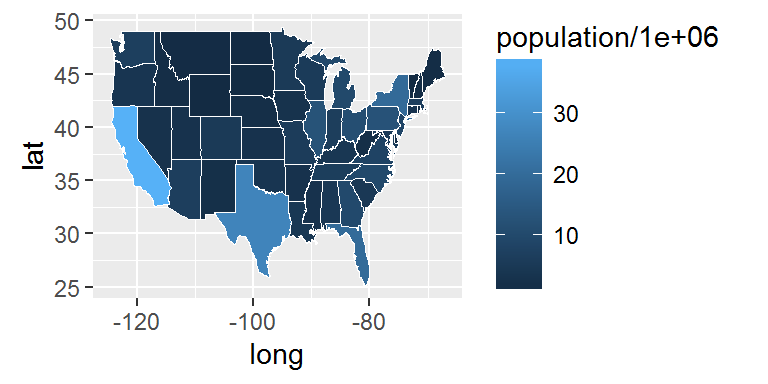Remarks

• Instead of using population, we use population/1000000. Each state is colored by population size (in millions) to make the legend easier to read.
• Border color (white) and line thickness (0.2) are specifically defined within this geom.

Once a map is created, it is often helpful to modify color schemes, determine how to address missing values (na.values) and formalize labels. Notice that we assigned the graph a name, p. This is particularly useful as we add new components to the graph.

p <- p + scale_fill_continuous(name="Population(millions)",
low = "lightgreen", high = "darkgreen",limits = c(0,40),
breaks=c(5,10,15,20,25,30,35), na.value = "grey50") +

labs(title="State Population in the Mainland United States")
p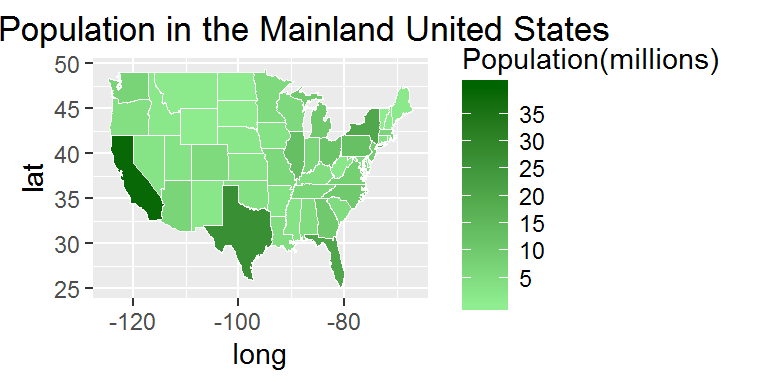Questions:

1. What two columns were added to the MainStates file when it was joined with the StatePopulation file?

2. Create a choropleth map showing state populations. Make the state borders purple with size = 1. Also change the color scale for state populations, with low populations colored white and states with high populations colored dark red.

3. Modify the graph and legend in Question 5) to show the log of populations instead of the population in millions. In this map, explain why you will need to set new limits and breaks. Hint: create a map without setting specific limits and breaks values. How does the graph change?

The following code provides just a few more examples of how each map can be customized. The ggplot2 website and the Data Visualization Cheat Sheet provides many additional detailed examples.

The following code modifies the previous graph by modifying the height and thickness of the legend and by adjusting the color, size and angle of the legend text.

p <- p + guides(fill = guide_colorbar(barwidth = 0.5, barheight = 10,
label.theme = element_text(color = "green", size =10, angle = 45)))
p

It is also possible to overlay two polygon maps. The code below creates county borders with a small line size and then adds a thicker line to represent state borders. The alpha = .3 causes the fill in the state map to be transparent, allowing us to see the county map behind the state map.

AllCounty <- map_data("county")
ggplot() + geom_polygon( data=AllCounty, aes(x=long, y=lat, group=group),
color="darkblue", fill="lightblue", size = .1 ) +

geom_polygon( data=MainStates, aes(x=long, y=lat, group=group),
color="black", fill="lightblue",  size = 1, alpha = .3)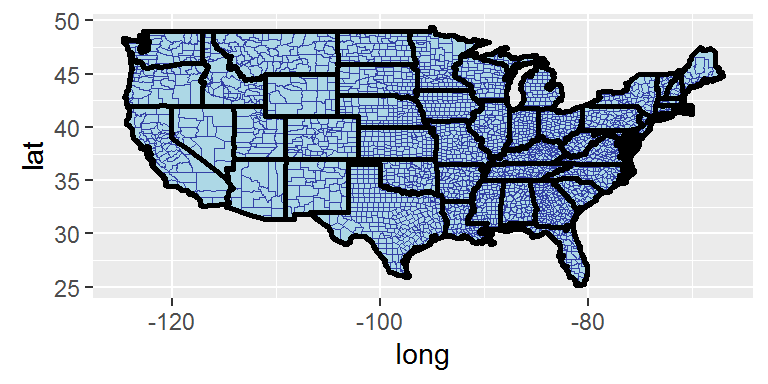4. Adding points to your choropleth map

The maps package also includes a us.cities file. The following code adds a point for each major city in the United States. Notice that the size of the point is determined by the population of that city.

#plot all states with ggplot
p <- p + geom_point(data=us.cities, aes(x=long, y=lat, size = pop)) +

scale_size(name="Population")
p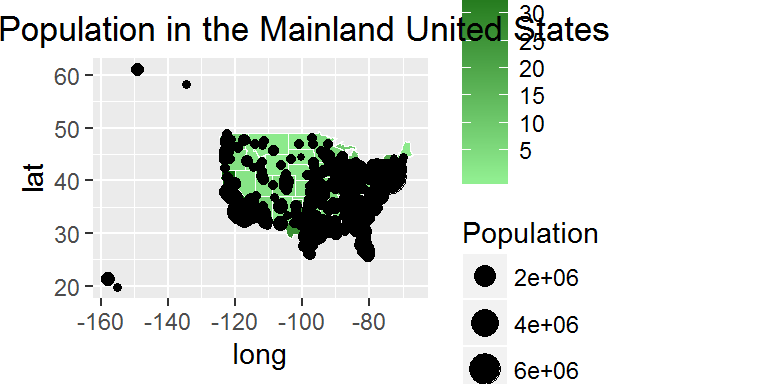us.cities2=arrange(us.cities, pop)
tail(us.cities2)
##                 name country.etc     pop   lat    long capital
## 1000 Philadelphia PA          PA 1439814 40.01  -75.13       0
## 1001      Phoenix AZ          AZ 1450884 33.54 -112.07       2
## 1002      Houston TX          TX 2043005 29.77  -95.39       0
## 1003      Chicago IL          IL 2830144 41.84  -87.68       0
## 1004  Los Angeles CA          CA 3911500 34.11 -118.41       0
## 1005     New York NY          NY 8124427 40.67  -73.94       0

It appears that the us.cities file includes cities in Hawaii and in Alaska. We will again us the dplyr package to eliminate these four cities and make a final map.

#plot all states with ggplot
MainCities <- filter(us.cities, long>=-130)
# str(us.cities)
# str(MainCities)

g <- ggplot()
g <- g + geom_polygon( data=MergedStates,
aes(x=long, y=lat, group=group, fill = population/1000000),
color="black", size = 0.2) +

scale_fill_continuous(name="State Population", low = "lightblue",
high = "darkblue",limits = c(0,40), breaks=c(5,10,15,20,25,30,35),
na.value = "grey50") +

labs(title="Population (in millions) in the Mainland United States")
g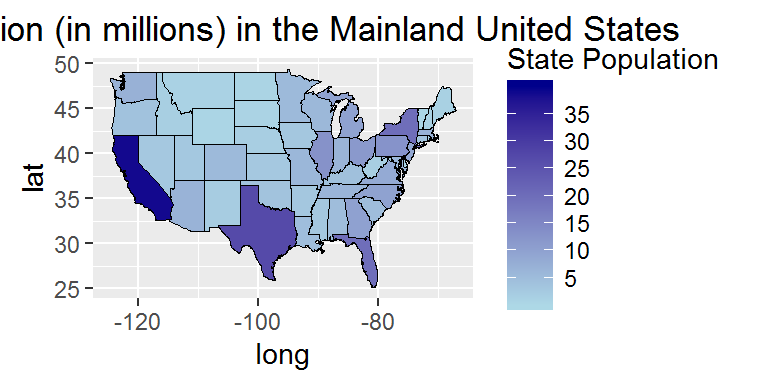g <- g + geom_point(data=MainCities, aes(x=long, y=lat, size = pop/1000000),
color = "gold", alpha = .5) + scale_size(name="City Population")
g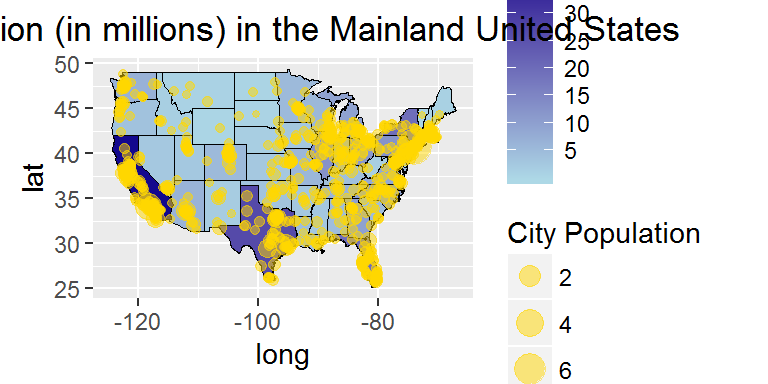# Zoom into a particular region of the plot
g  <- g + coord_cartesian(xlim=c(-80, -65), ylim = c(38, 46))
g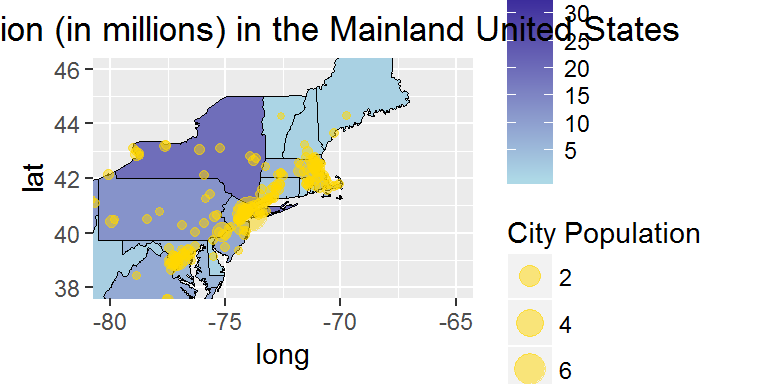5. On your own

• Restrict the states and all.cities files to only a few contiguous states, such as New York, Vermont, New Hampshire, Massachusetts, Rhode Island, and Connecticut. You could use the following code:
NewStates <- filter(MainStates,region ==  "new york" | region ==
"vermont" | region ==  "new hampshire" | region ==
"massachusetts" | region ==  "rhode island" |
region ==  "connecticut" )
• Create a map of these states and color each state by the number of electorial votes in that state.
• Add a point to represent each city in these states.
• Color the cities so that state capitals are clearly differentiated from other cities. In the geom_point you may want to use the following code:
g <- g + geom_point(data=MainCities, aes(x=long, y=lat,
size = pop/1000000, color=factor(capital), shape = factor(capital)),
alpha = .5) +

scale_size(name="City Population")